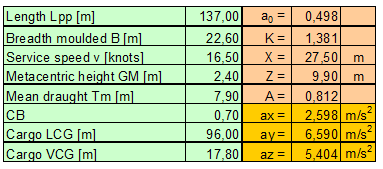6. Interpretation of „on deck high“ [German version]

The higher the level of stowage above the waterline of a vessel the greater are the expected longitudinal and transverse forces to a cargo item. The desired handiness of the Annex 13 securing assessment method and its applicability to a certain range of ship sizes had caused the introduction of four distinguished stowage levels instead of using the specific vertical position of the cargo unit in question. While the stowage levels „lower hold“, „tween deck“ and „on deck low“ are easy to identify by users, the level of „on deck high“ has quite often provoked disputes among parties.

With extremely large cargo items the category „on deck high“ appears to be ambiguous. In order to avoid uncertainties in the determination of transverse and longitudinal accelerations in such cases, it is recommended, to use the original mathematical model, which has been the basis for the Annex 13 acceleration tables. This model may be less convenient to use, but is worth to be programmed, e.g. in a suitable spread sheet. Classification societies are prepared to assess and certify such programs. An outline of the relevant mathematical model is shown below and should be included in an appendix to the Annex 13.

The longitudinal, transverse and vertical accelerations acting on a cargo unit may be obtained alternatively by the set of formulas as follows:

ax: longitudinal acceleration (gravity component of pitch included)

ay: transverse acceleration (gravity component of roll included)

az: vertical acceleration (component due to static weight not included)

c1: correction factor for navigation area, taken as 1.0 = worldwide in the Annex 13

c2: correction factor for season, taken as 1.0 = whole year in the Annex 13

c3: correction factor for 25 navigation days, c3 = 0.6 + 0.1 log1025 = 0.74 in the Annex 13

therein:

L = length of ship [m] (Lpp in the Annex 13)

B = breadth of ship [m] (moulded breadth in the Annex 13)

GM = metacentric height of the vessel [m]

Cb = block coefficient of ship

x = longitudinal distance from amidships to calculating point, positive forward [m]

z = vertical distance from actual waterline to calculating point, positive upward [m]

v = service speed [knots]

g = gravity acceleration = 9.81 m/s2